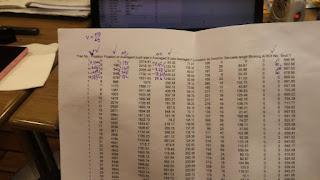### how to calculate acceleration?

Prof. Tsai;

I got problem in calculating the acceleration.
I know how to obtain  velocity within certain line (eg. dot 1 to dot 2; dot 2 to dot 3)
Since we have the x position and the time stamp (time onset, not the fixation duration, right?).

From the physics I know if I need to obtain acceleration, I need to differentiate △v by △T
But the question is:

what is the △T here? Is it the one obtained from the first delta T ?
I got a diagram for you.

Noted,
Howard

--

Battery, Browsers, Scenarist